# 以 dy/dx 来看导数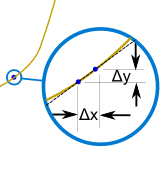……导数表达在任何一点的改变速度（叫变化率）。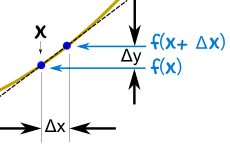y = f(x)

## 一、加 Δx

y + Δy = f(x + Δx)

 从： y + Δy = f(x + Δx) 减： y = f(x) 结果： y + Δy − y = f(x + Δx) − f(x) 简化： Δy = f(x + Δx) − f(x)

## 三、变化率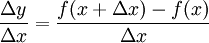## 四、把 Δx 缩小到接近于 0

Δxdx## 用个函数来试试看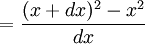f(x) = x2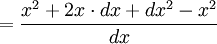展开 (x+dx)2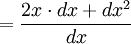简化 (x2-x2=0)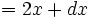简化分数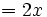dx 趋近 0